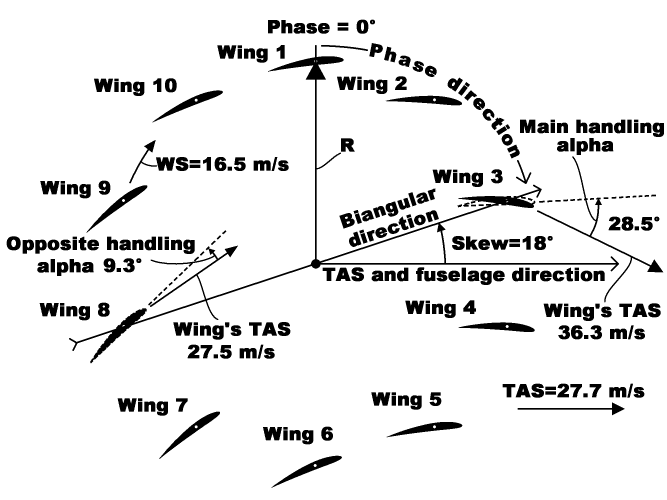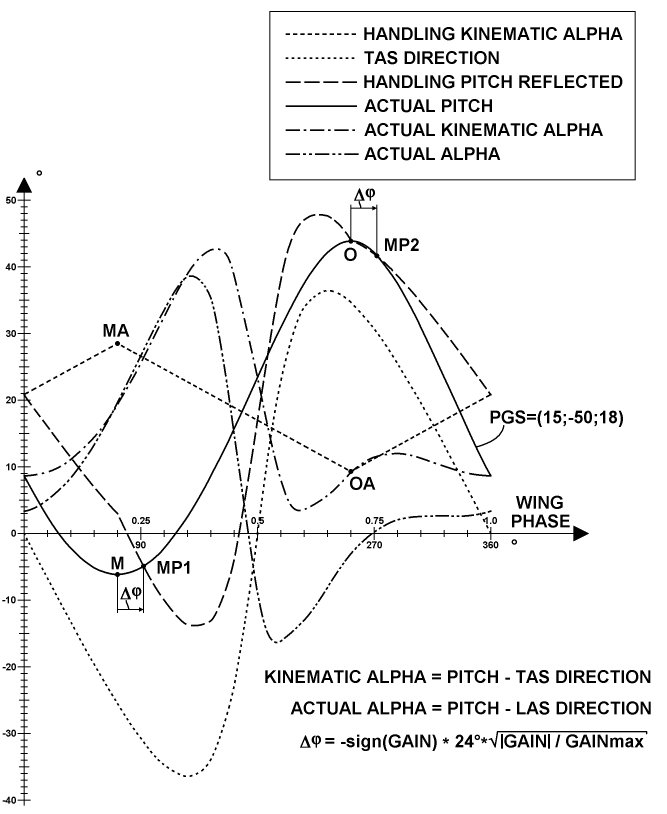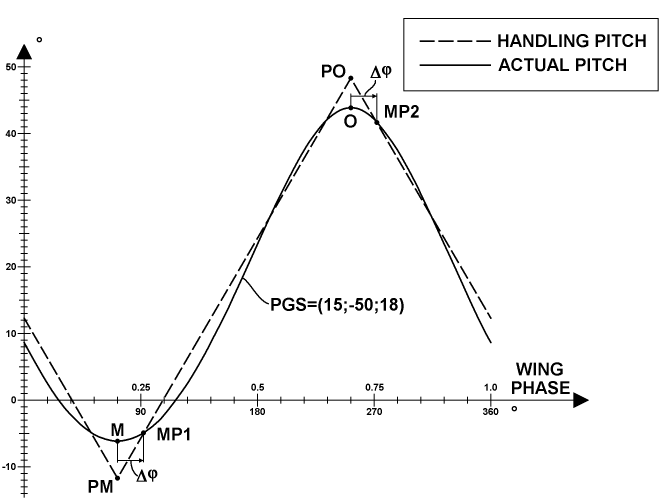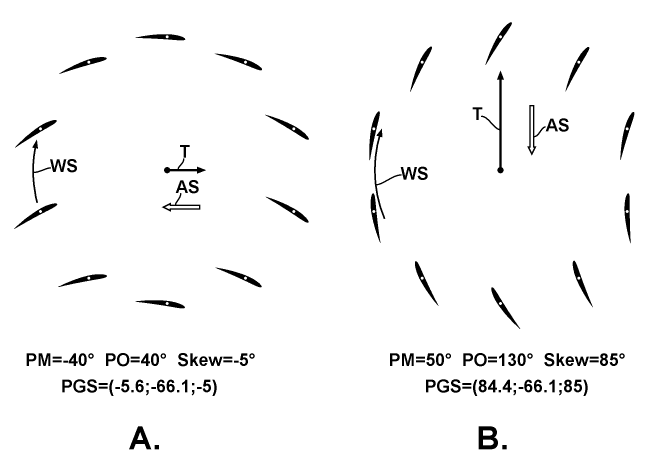﻿ Liftoplane: Biangular handling

# Biangular handling

A high-level handling mode of the liftoplane is referenced as biangular handling. The diagram below explains it.The main idea there is: the high level handling should have direct relation with angles of attack of wings in two opposite points in some direction. The direction is selected as being pointed by the Skew angle of the PGS-state, and so it is equal to the gain direction. And so there are two AoAs for the main and opposite points. In aerodynamics AoA is usually referenced also as angle alpha. And so I reference those two angles as the main handling alpha and the opposite handling alpha, and they are reflected on the diagram, which utilizes same PGS-state as the diagram of explanation of the PGS-state. Those two angles are represented for Wing 3 and Wing 8. Main difference of the handling from the PGS-state is using of an airflow condition connected to those angles by a way, having them aren't depend from changing of parameters of the flow condition itself. For example, after providing particular values of such biangular angles to some flight software routine, they will be constant for any change of the airspeed and for any change of the winding speed of rotor. The constant behavior will be ensured by a correspondent change of the P and G components of PGS-state from the routine upon measuring the changes of the airspeed and the rotor's winding speed. The diagram presents example of the relation, picturing the winding speed WS, the true aerodynamic speed TAS of entire aircraft, which is parallel to the fuselage in the mode of handling, and two particular TAS vectors for those Wing 3 and Wing 8. The angles of attack relative to those two TAS vectors imply two related pitches for those two wings. Such positions of those wings and directions of their chords are depicted on the diagram by the dot-line. And they aren’t equal to the actual performed pitches. This differencing feature reflects a special correction of asymmetry of the pitch variation imposed by the gain to the main and opposite points. The correction is significant for high gain values and it is near to zero for low gain. Additionally, for case of existing of some remained error angle between fuselage and the TAS vector, the error angle should be in consideration upon handling and should be used for a correction of resulted PGS-state.The chart above represents a plot of distributions of all related angular components participated in the biangular handling over entire wing positions of the rotor for example from the previous diagram. At first, there are values of HANDLING CINEMATIC ALPHA. The word "cinematic" means: the reference flow is simple vectorial sum of the TAS of aircraft and the cinematic speed component of particular wing for its particular phase. It doesn’t include dynamic perturbations of the airflow from actual vorticity distribution over entire rotor. The exclusion means: any handling parameters should be free from all dynamic components containing some uncertainty errors, for the steady referencing. And those biangular values are simple cinematic parameters, although some level of uncertainty can still exist upon measuring the TAS. The primary handling distribution is selected to be simple linear between the main alpha (MA) and the opposite alpha (OA). At second, there are values of the TAS DIRECTION itself. At third, there are values of HANDLING PITCH REFLECTED from the first relation for the cinematic alpha referenced at bottom of the plot. These last values correspond to the pitches depicted by the dot line on the previous diagram. At fourth, there are values of ACTUAL PITCH resulted from the PGS-state. Those two kinds of pitches are connected by using two match points: MP1, which is shifted from the main direction M of the PGS-state on angle Δφ, and MP2, which shifted from the opposite direction O of the PGS-state on same angle. The formula for the shifting angle is represented in the third equation at bottom of the plot. It uses the Gain itself and is simple best-rounded approximation for fitting the actual pitch distribution to the desired handling pitch distribution, which I found through numerical experimentations. The flight software routine uses in the equation a Gain value from previous cycle of its servicing, since the Gain is also component of the target result for fitting these match points. At fifth, there are values of ACTUAL CINEMATIC ALPHA, recovered from the first relation referenced at bottom of the plot. And at sixth, there are values of ACTUAL ALPHA, recovered from the second relation referenced at bottom of the plot. The second relation uses LAS instead of the TAS. For obtain the LAS, perturbation of the base flow by the vorticity were split on the inflow component and the interference distribution component , as result of numerical simulation. And the entire result corrects the TAS to the LAS.There is also a simplified variant of the biangular handling mode, which ignores the airflow condition, for rare use, which I reference as biangular pitch handling. The chart above represents a plot of distributions of pitches over entire wing positions of the rotor for the case, utilizing the same PGS-state as for the biangular alpha handling. The ACTUAL PITCH distribution is depicted together with values of HANDLING PITCH, which is also selected to be simple linear between the main pitch (PM) and the opposite pitch (PO) as for the alpha mode. The matching between these two kinds of pitches is performed by exactly same algorithm as for the alpha mode.The chart above represents two diagrams designated as A. and B. for particular using the biangular pitch handling in a such named "propelling" mode for runway acceleration and for trying a vertical takeoff respectively. These diagrams depict relations of the wing articulation, thrust T, winding speed WS and airspeed AS. The main feature of this mode is: the P value of PGS is almost same as the S value and is following for a changing of the other. Here the PM and PO values are used only for a reference. Also the thrust force for the second case still isn't enough to accomplish it. Detailed comparative analysis with an equivalent rotor, operating in the curtate mode, indicates lower thrust then represented rotor, but also it has a much lower external moment and consumed power. And if enough power for takeoff will be provided for both kinds of rotors, the rotor with curtate movement will spend much less power with much lower external moment, but will have a winding speed much higher then represented rotor, so too high centrifugal forces will damage it, before this condition will met.